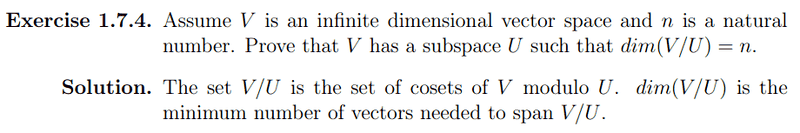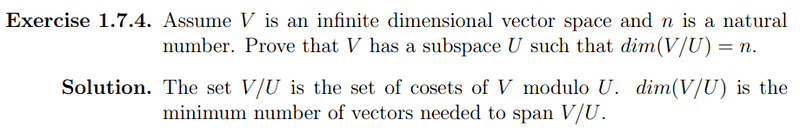# Showing an infinite dimensional vector space has an arbitrary dimensional quot. spaceI'm trying to understand this problem. Let's take an infinite dimensional vector space, say $\mathbb{R}^2$ and let $n = 4$. This problem states we can find a subspace $U$ such that dim(\mathbb{R}^2/U) = 4$. Well, one subspace of$\mathbb{R}^2$is $U = \{(x, y) : x*y \geq 0\}$ (i.e. the first and third quadrant). So $\mathbb{R}^2 / U = \{v + U : v \in \mathbb{R}^2\}$. But that means $\mathbb{R}^2 / U = \mathbb{R}^2$, right? ## Answers and Replies Dick Science Advisor Homework HelperI'm trying to understand this problem. Let's take an infinite dimensional vector space, say $\mathbb{R}^2$ and let $n = 4$. This problem states we can find a subspace$U$such that dim(\mathbb{R}^2/U) = 4$. Well, one subspace of $\mathbb{R}^2$ is $U = \{(x, y) : x*y \geq 0\}$ (i.e. the first and third quadrant). So $\mathbb{R}^2 / U = \{v + U : v \in \mathbb{R}^2\}$. But that means $\mathbb{R}^2 / U = \mathbb{R}^2$, right?

That's pretty much all wrong so far. Just because a vector space has an infinite number of vectors doesn't make it infinite dimensional. If the scalar field is R, R^2 isn't infinite dimensional, what is the dimension? U isn't a subspace, why not? Try and review some definitions.

That's pretty much all wrong so far. Just because a vector space has an infinite number of vectors doesn't make it infinite dimensional. If the scalar field is R, R^2 isn't infinite dimensional, what is the dimension? U isn't a subspace, why not? Try and review some definitions.

I feel really stupid for claiming that $R^2$ is infinite dimensional. Let me try again. Let $V$ be the set of all polynomials with coefficients in $R$. Let $n = 4$.

We have to find a subspace of V, U such that V / U has degree 4.

But V / U is the set of all cosets of U in V. Let's say U is the set of all cubic polynomials. Wouldn't V / U = v + U = V?

Dick
Homework Helper

I feel really stupid for claiming that $R^2$ is infinite dimensional. Let me try again. Let $V$ be the set of all polynomials with coefficients in $R$. Let $n = 4$.

We have to find a subspace of V, U such that V / U has degree 4.

But V / U is the set of all cosets of U in V. Let's say U is the set of all cubic polynomials. Wouldn't V / U = v + U = V?

Well, no it wouldn't exactly be V. But it would be infinite dimensional, if that's what you mean. Let's skip the 'infinite dimensional' part for a bit. Suppose you are given the ten dimensional space V=R^10 and you want to show me that there is a subspace U of V such that V/U has dimension 4. How would you do that?

I would let $U = \{(a, b, c, d, e, f, 0, 0, 0, 0) : a, b, c, d, e, f \in \mathbf{R}\}$ Because I think there's a relationship where dim(V) = dim(U) + dim(V/U). Based on that equation, dim(V/U) = 4.

But that doesn't make sense to me because the set of all cosets of U in V
would be all (a, b, c, d, e, f, 0, 0, 0, 0) + V which would be V itself, right?

Dick
Homework Helper

I would let $U = \{(a, b, c, d, e, f, 0, 0, 0, 0) : a, b, c, d, e, f \in \mathbf{R}\}$ Because I think there's a relationship where dim(V) = dim(U) + dim(V/U). Based on that equation, dim(V/U) = 4.

But that doesn't make sense to me because the set of all cosets of U in V
would be all (a, b, c, d, e, f, 0, 0, 0, 0) + V which would be V itself, right?

The union of all of the vectors in all of the cosets is V, yes. But that's not the point. V/U is a set of cosets, not a set of vectors. (a, b, c, d, e, f, 0, 0, 0, 0) is one coset, (a, b, c, d, e, f, 1, 0, 0, 0) is another coset. (a, b, c, d, e, f, 2, 0, 0, 0) is another coset. (a, b, c, d, e, f, 0, 1, 0, 0) is yet another coset. Each coset is itself a six dimensional space. The SET OF COSETS is 4 dimensional. Does that make sense?